# GMAT Math : DSQ: Calculating the volume of a cylinder

## Example Questions

### Example Question #45 : Rectangular Solids & Cylinders

What is the volume of the cylinder?

Statement 1: the cylinder has a radius of 3

Statement 2: the cylinder has a height of 4

BOTH statements TOGETHER are sufficient, but NEITHER statement ALONE is sufficient.

Statements 1 and 2 TOGETHER are NOT sufficient.

Statement 2 ALONE is sufficient, but statement 1 is not sufficient.

EACH statement ALONE is sufficient.

Statement 1 ALONE is sufficient, but statement 2 is not sufficient.

BOTH statements TOGETHER are sufficient, but NEITHER statement ALONE is sufficient.

Explanation:

The formula for the volume of a cylinder is: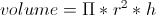Therefore we need both Statement 1 and 2 to find the volume, so both statements together are sufficient, but neither statement alone is sufficient.

### Example Question #46 : Rectangular Solids & Cylinders

Give the radius of a cylinder with volume 1,000 cubic inches.

1) Its height is 40 inches.

2) The area of its base is 25 square inches.

Statement 1 ALONE is sufficient to answer the question, but Statement 2 ALONE is not sufficient.

EITHER Statement 1 or Statement 2 ALONE is sufficient to answer the question.

BOTH statements TOGETHER are sufficient to answer the question, but NEITHER statement ALONE is sufficient to answer the question.

Statement 2 ALONE is sufficient to answer the question, but Statement 1 ALONE is not sufficient.

BOTH statements TOGETHER are NOT sufficient to answer the question.

EITHER Statement 1 or Statement 2 ALONE is sufficient to answer the question.

Explanation:

The two statements are actually equivalent; ifis its volume,is the area of its base, andis its height, then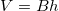, or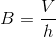. So if we know the first statement, that is,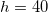, then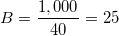, which is the second statement.

To find the radius, use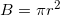, or, equivalently,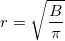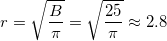The answer is that either statement alone is sufficient to answer the question.

### Example Question #47 : Rectangular Solids & Cylinders

A factory makes two sizes of cylindrical barrels. How many small cylindrical barrels full of liquid would it take to fill a large barrel?

Statement 1: A large cylindrical barrel is twice as high as a small cylindrical barrel.

Statement 2: A large cylindrical barrel is three times as wide as a small cylindrical barrel.

BOTH statements TOGETHER are sufficient to answer the question, but NEITHER statement ALONE is sufficient to answer the question.

BOTH statements TOGETHER are NOT sufficient to answer the question.

Statement 1 ALONE is sufficient to answer the question, but Statement 2  ALONE is NOT sufficient to answer the question.

EITHER statement ALONE is sufficient to answer the question.

Statement 2 ALONE is sufficient to answer the question, but Statement 1  ALONE is NOT sufficient to answer the question.

BOTH statements TOGETHER are sufficient to answer the question, but NEITHER statement ALONE is sufficient to answer the question.

Explanation:

The question is essentially this: if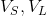are the volumes of the barrels, then find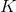such that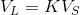,

or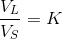Equivalently,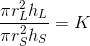orThis means that knowing both the ratio of the heights and the ratio of the radii (and subsequently, the widths) is necessary and sufficient. Therefore, you need both statements, and both together are sufficient.

### Example Question #48 : Rectangular Solids & Cylinders

Which of Cylinder 1 and Cylinder 2, if either, has the greater volume?

Statement 1: The height of Cylinder 1 is equal to the radius of the base of Cylinder 2.

Statement 2: The height of Cylinder 2 is equal to twice the radius of the base of Cylinder 1.

BOTH statements TOGETHER are insufficient to answer the question.

Statement 1 ALONE is sufficient to answer the question, but Statement 2 ALONE is NOT sufficient to answer the question.

EITHER statement ALONE is sufficient to answer the question.

BOTH statements TOGETHER are sufficient to answer the question, but NEITHER statement ALONE is sufficient to answer the question.

Statement 2 ALONE is sufficient to answer the question, but Statement 1 ALONE is NOT sufficient to answer the question.

BOTH statements TOGETHER are insufficient to answer the question.

Explanation:

Assume both statements. We show that insufficient information is provided using two cases.

Case 1: Cylinder 1 has height 10 and radius 20. Then Cylinder 2 has height 40 and radius 10.

The volume of Cylinder 1 is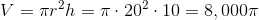The volume of Cylinder 2 is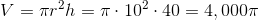Case 2: Cylinder has height 40 and radius 10. Then Cylinder 2 has height 20 and radius 40.

The volume of Cylinder 1 isThe volume of Cylinder 2 is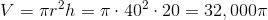In one case, Cylinder 1 had greater volume; in the other, Cylinder 2 did. This makes the two statements insufficient.

### Example Question #49 : Rectangular Solids & Cylinders

How many identical cans can be packed in a certain box?

(1) The box iscentimeters wide andcentimeters high.

(2) Each can iscentimeters high.

C: BOTH statements TOGETHER are sufficient, but NEITHER statement ALONE is sufficient

A: Statement (1) ALONE is sufficient, but statement (2) ALONE is not sufficient

B: Statement (2) ALONE is sufficient, but statement (1) ALONE is not sufficient

E: Statements (1) and (2) TOGETHER are not sufficient

D: EACH statement ALONE is sufficient

E: Statements (1) and (2) TOGETHER are not sufficient

Explanation:

(1) does not give us any information about the cans' dimension or the length of the box, so it is not sufficient alone.

(2) does not give us box dimensions or any information about the cans' diameter, so it is not sufficient alone.

Both statement taken together give more information but we still dont have complete dimensions for the box or each can.

Therefore the right answer is E.

### Example Question #50 : Rectangular Solids & Cylinders

Jenkins has a poster tube which he is using to carry his posters to college.

I) The poster tube has a volume of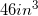.

II) The poster tube isinches long.

What is the radius of the poster tube?

Either statement alone is sufficient to answer the question.

Statement II is sufficient to answer the question, but statement I is not sufficient to answer the question.

Statement I is sufficient to answer the question, but statement II is not sufficient to answer the question.

Both statements are necessary to answer the question.

Both statements are necessary to answer the question.

Explanation:

Recall the formula for volume of a cylinder: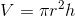Statement I gives us V

Statement II gives us h

We can then use both of them to find our "r." We cannot do it without both of them.

### Example Question #1 : Dsq: Calculating The Volume Of A Cylinder

How much water, in cubic feet, can a cylindrical water tank whose bases have radius 6 feet hold?

Statement 1: The lateral area of the tank is 125.66 square yards.

Statement 2: The tank is 30 feet high.

BOTH statements TOGETHER are sufficient to answer the question, but NEITHER statement ALONE is sufficient to answer the question.

EITHER statement ALONE is sufficient to answer the question.

BOTH statements TOGETHER are insufficient to answer the question.

Statement 1 ALONE is sufficient to answer the question, but Statement 2 ALONE is not sufficient to answer the question.

Statement 2 ALONE is sufficient to answer the question, but Statement 1 ALONE is not sufficient to answer the question.

EITHER statement ALONE is sufficient to answer the question.

Explanation:

We are given the radius; if we know the height, we can use the formulato calculate the volume of the tank.

The second statement gives us that the tank is 30 feet high. But the first statement gives us the way to find the height by using the lateral area formula.

First we have to convert square yards to square feet by multiplying by 9.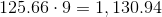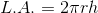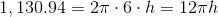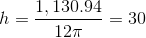Either way, we now have both radius and height, and we can find the volume: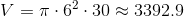The answer is that either statement alone is sufficient to answer the question.

Tired of practice problems?

Try live online GMAT prep today.